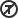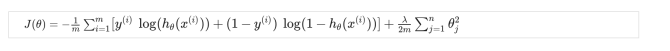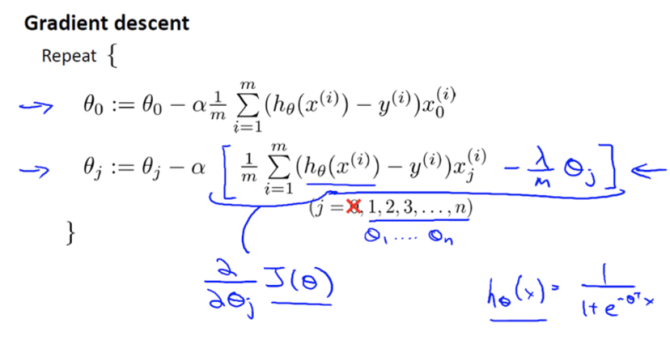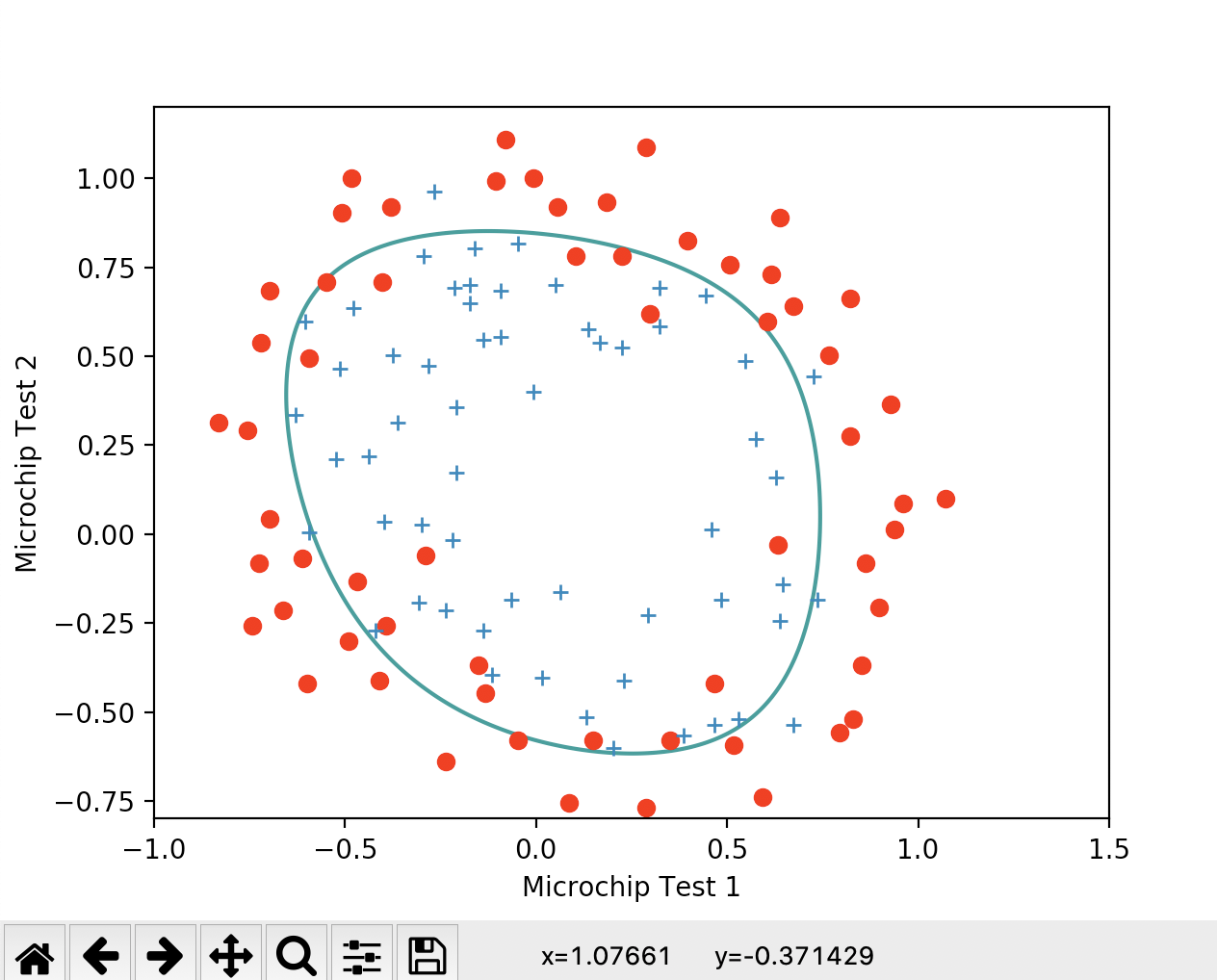# 机器学习菜鸟的学习笔记（14）

• Python
• 机器学习原创

### 写在前面

，其实也并不算复杂，所见即所得，所得就把它写出来。

### 正则化逻辑回归的实现

#### 文件数据

with open(filename, 'r') as f:
columns = ['t1', 't2', 'value']
save_list.append([float(row), float(row), int(row)])
df = pd.DataFrame(save_list, columns=columns)


plt.plot(data_when_zero['t1'], data_when_zero['t2'], 'ro')
plt.plot(data_when_one['t1'], data_when_one['t2'], '+')
plt.xlabel('Microchip Test 1')
plt.ylabel('Microchip Test 2')#### 数据处理

 def mapFeature(x1, x2):
power = 6
data = {}
for i in np.arange(power + 1):
for p in np.arange(i + 1):
data["f{}{}".format(i - p, p)] = np.power(x1, i - p) * np.power(x2, p)

return pd.DataFrame(data)


#### 正则化# compute cost and grad
def costFunctionReg(theta_in, XX, yy):
lambda_in = 1
m = len(yy)
hypo = sigmoid(XX @ theta_in)
# print(sum(np.power(theta_in[1:len(theta_in)], 2)))
J = 1 / m * sum(-yy.T @ np.log(hypo) - (1 - yy).T @ (np.log(1 - hypo)))+(lambda_in / (2 * m) * sum(np.power(theta_in[1:len(theta_in)], 2)))
return J


### 结果展示0

0

0